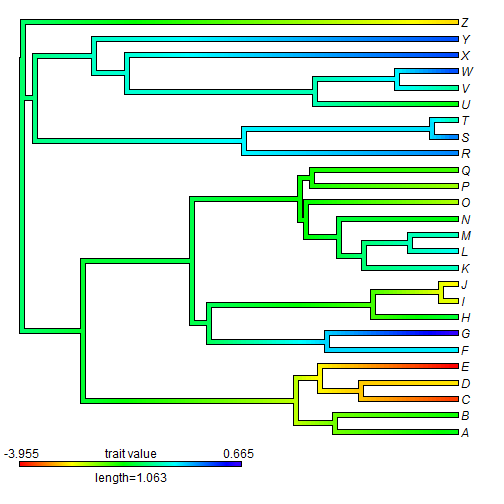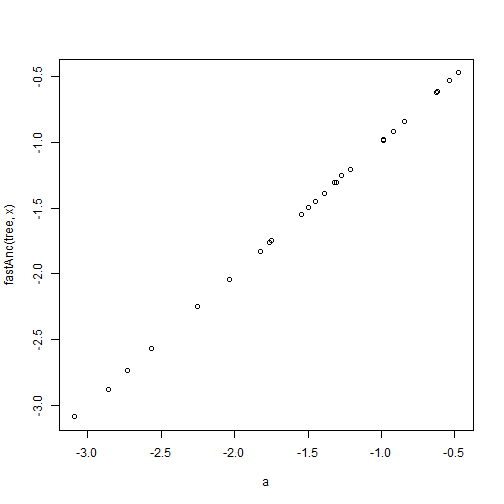## Friday, September 4, 2015

### 'Extracting' internal node values from an object of class "contMap"

A phytools user asked today if there is some way to 'extract' the reconstructed trait values from internal nodes in an object of class `"contMap"`.

In fact, this is possible. (Although, as I'll explain below, the circumstances in which we want to do this are probably pretty limited.)

``````## load packages
library(phytools)
## simulate tree & data
x<-fastBM(tree<-pbtree(n=26,tip.label=LETTERS))
## build object of class "contMap"
obj<-contMap(tree,x,plot=FALSE)
obj
``````
``````## Object of class "contMap" containing:
##
## (1) A phylogenetic tree with 26 tips and 25 internal nodes.
##
## (2) A mapped continuous trait on the range (-3.954532, 0.664747).
``````
``````plot(obj)
````````````## now let's first get the indices in obj\$cols corresponding
## to the *end* of each edge
ii<-c(as.numeric(names(obj\$tree\$maps[])),
sapply(obj\$tree\$maps,function(x) as.numeric(names(x)[length(x)])))
## find the trait values corresponding to each index
a<-setNames(ii/(length(obj\$cols)-1)*diff(obj\$lims)+obj\$lims,
c(obj\$tree\$edge[1,1],obj\$tree\$edge[,2]))
a
``````
``````##          27          28          29          30           1           2
## -1.31692381 -1.54788773 -2.56412896 -2.25001804 -1.70494319 -1.81118659
##          31          32           3           4           5          33
## -2.85976278 -3.09072670 -3.68661360 -2.97062546 -3.94067391 -1.45088288
##          34          35           6           7          36           8
## -1.39083227 -0.47621515 -0.60093567  0.64165021 -2.03753123 -1.54326845
##          37           9          10          38          39          40
## -2.72580371 -2.73504226 -2.86900134 -1.76499381 -1.75113598 -1.49707567
##          41          11          42          12          13          14
## -1.27073103 -0.92890443 -0.98433577 -0.73951402 -0.95200082 -1.71418175
##          15          43          16          17          44          45
## -2.49022051 -1.82042515 -2.42555061 -2.03291196 -1.30768526 -1.21068041
##          46          18          47          19          20          48
## -0.62403206 -0.13438856 -0.53626577 -0.12976928 -0.92890443 -0.91966587
##          49          50          21          51          22          23
## -0.84113814 -0.98433577 -1.70956247 -0.61941278 -1.07672134  0.09657536
##          24          25          26
##  0.12891031  0.10119464 -3.00296041
``````
``````## pull out only the node states
a<-a[as.character(1:tree\$Nnode+Ntip(tree))]
a
``````
``````##         27         28         29         30         31         32
## -1.3169238 -1.5478877 -2.5641290 -2.2500180 -2.8597628 -3.0907267
##         33         34         35         36         37         38
## -1.4508829 -1.3908323 -0.4762152 -2.0375312 -2.7258037 -1.7649938
##         39         40         41         42         43         44
## -1.7511360 -1.4970757 -1.2707310 -0.9843358 -1.8204252 -1.3076853
##         45         46         47         48         49         50
## -1.2106804 -0.6240321 -0.5362658 -0.9196659 -0.8411381 -0.9843358
##         51
## -0.6194128
``````

Now, the reason we don't really need to do this is because the node states are going to be the same as those estimated using functions such as `fastAnc` or `anc.ML`. (They will be slightly different just due to the fact that the color mapping is finely discretized across the edges & nodes of the tree.)

For instance:

``````plot(a,fastAnc(tree,x))
``````